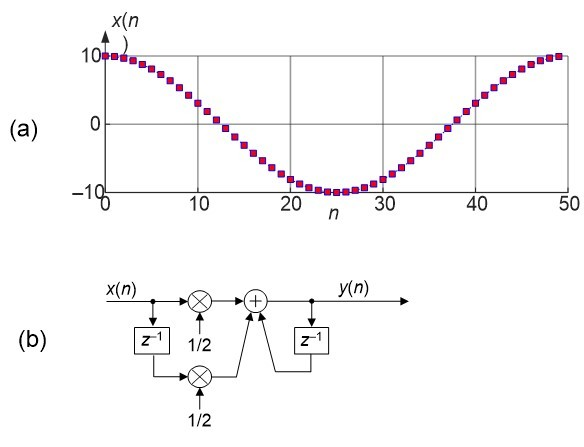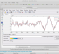(So far, 57 people got it right out of 213 for a success rate of 26%)

(Thank you to Rick Lyons, author of the top-selling book Understanding Digital Signal Processing, for submitting this Quiz Question.)

Figure 1(a) is x(n), a 50-sample sequence of exactly one cycle of a cosine wave.Using the Trapezoidal Rule network in Figure 1(b), which of the following most closely estimates the discrete definite integral of the Figure 1(a) cosine?

Pick one:
-0.02
+0.02
-10
+10

[ - ]
Comment by February 20, 2020Hello Rick,

If I use the trapz() function with octave software, result is -9.9606. But, If I use the Trapezoidal Rule network in Figure 1(b), result depend of the initial value : y(0)=0 gives a good result but with y(0)=5 (this is right value with y(n)=y(n-1)+0.5(x(n)+x(n-1))) result becomes -4.9606.

My equation is certainly wrong, could you explain to me how the trapz () function works ?

Thank you very much,

Fabrice.

[ - ]
Comment by February 21, 2020Hi Fabrice. I just now saw your Comment.

Your Octave trapz() result agrees with MATLAB's trapz() result.

When its input is a simple sequence of numbers MATLAB's trapz() command computes a result that is equal to the sum of all the input samples minus half the sum of the first input sample plus the last input sample. In my cosine wave example that result is -9.9606.

I realize now that I should not have included the Fig. 1(b) network in my Quiz Question. Including that network causes unexpected problems that you experienced. That network is the implementation of the z-transform transfer function of the traditional trapezoidal difference equation given in most DSP textbooks.

That traditional trapezoidal difference equation is:

y(n) = 0.5x(n) + 0.5x(n-1) + y(n-1),

and its z-transform transfer function is:

H(z) = Y(z)/X(z) = [0.5 + 0.5z^(-1)]/[1 –z^(-1)].

The surprising problem with implementing the z-transform transfer function of the traditional trapezoidal difference equation (that is, the network in Fig. 1(b)) is that the result is in error by x(0)/2 (half the first sample value). Processing my cosine wave example using the Fig. 1(b) network produces a result of -4.9606, as you found out! The network's -4.9606 result is the correct -9.9606 result plus an error of x(0)/2 = 10/2 = 5.

The point of my Quiz Question was that we expect the definite integral of a single cycle of a cosine wave to be zero (or nearly zero). But that is not the case when the Trapezoidal Rule integration algorithm is used.

If I haven't bored you to death by now, and you enjoy DSP as much as I do, here are a few things you can experiment with:

 Compute the definite integral of my original 50-sample single-cycle cosine wave using the Rectangular Rule integration method.

 Create 50-sample cosine wave containing one more sample than an exact single-cycle cosine using:

x = 10*cos(2*pi*n*1/(N-1))

and compute the new x's definite integral using the Trapezoidal Rule.

 Compute the definite integral of Step 's new cosine wave using the Rectangular Rule integration method.

[ - ]
Comment by February 22, 2020Hi Rick,

Fabrice

[ - ]
Comment by February 29, 2020Hi,

I got

-9.960573506572363

both with  implementation of Fİg 1(b) (I took y = 0) and Python-numpy function of numpy.trapz and

i)

import numpy as np
i = np.arange(50)
x = np.cos(2*np.pi *i/50)*10
y = np.zeros(50)
for n in range(1,50):
y[n] = y[n-1] + 0.5* ( x[n] + x[n-1])
print(y[-1])

-9.960573506572363

ii)

np.trapz(x)

-9.960573506572409

[ - ]
Comment by February 29, 2020Hi Omersayli. Thanks for sharing your computed result with us.

[ - ]
Comment by February 29, 2020Thanks Rick,

I think, for https://en.wikipedia.org/wiki/Trapezoidal_rule, we need to set y = 0 along with Fİg 1(b). That solves the ambiguity you have discussed above with Fabrice.

That is because Trapezoidal rule requires f(a) and f(b) (two points) seperated by a step distance - (b-a)*0.5*(f(a)+f(b)). Hence calculating y from single x value is meaningless at the start. Setting y = 0 along with Fİg 1(b). solves the ambiguity for trapezoidal integration with this network.

Omer

[ - ]
Comment by March 1, 2020Hi Omer. Yes, you are correct. Another way to describe how to implement my Fig. 1(b) network operate properly is as follows: Apply the first x(0) sample to the network but set y(0) equal to zero. Then perform the two data shift operations and begin applying the x(1) -to- x(N-1) input samples to the network and compute the y(1) -to- y(N-1) output samples. The y(N-1) output sample will be the correct Trapezoidal Rule integration result.

To post reply to a comment, click on the 'reply' button attached to each comment. To post a new comment (not a reply to a comment) check out the 'Write a Comment' tab at the top of the comments.